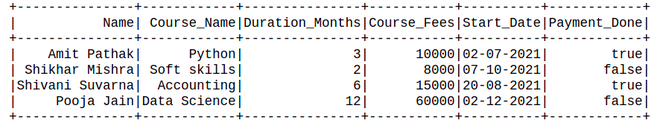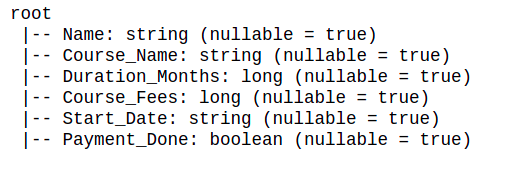Related Articles

# How to Change Column Type in PySpark Dataframe ?

• Last Updated : 18 Jul, 2021

In this article, we are going to see how to change the column type of pyspark dataframe.

Creating dataframe for demonstration:

## Python

 `# Create a spark session``from` `pyspark.sql ``import` `SparkSession``spark ``=` `SparkSession.builder.appName(``'SparkExamples'``).getOrCreate()`` ` `# Create a spark dataframe``columns ``=` `[``"Name"``, ``"Course_Name"``,``           ``"Duration_Months"``,``           ``"Course_Fees"``, ``"Start_Date"``,``           ``"Payment_Done"``]``data ``=` `[``    ``(``"Amit Pathak"``, ``"Python"``, ``3``,``     ``10000``, ``"02-07-2021"``, ``True``),``    ``(``"Shikhar Mishra"``, ``"Soft skills"``,``     ``2``, ``8000``, ``"07-10-2021"``, ``False``),``    ``(``"Shivani Suvarna"``, ``"Accounting"``,``     ``6``, ``15000``, ``"20-08-2021"``, ``True``),``    ``(``"Pooja Jain"``, ``"Data Science"``, ``12``,``     ``60000``, ``"02-12-2021"``, ``False``),``]``course_df ``=` `spark.createDataFrame(data).toDF(``*``columns)`` ` `# View the dataframe``course_df.show()`

Output:Let’s see the schema of dataframe:

## Python

 `# View the column datatypes``course_df.printSchema()`

Output:## Method 1: Using DataFrame.withColumn()

The DataFrame.withColumn(colName, col) returns a new DataFrame by adding a column or replacing the existing column that has the same name.

We will make use of cast(x, dataType) method to casts the column to a different data type. Here, the parameter “x” is the column name and dataType is the datatype in which you want to change the respective column to.

Example 1: Change datatype of single columns.

## Python

 `# Cast Course_Fees from integer type to float type``course_df2 ``=` `course_df.withColumn(``"Course_Fees"``, ``                                  ``course_df[``"Course_Fees"``]``                                  ``.cast(``'float'``))``course_df2.printSchema()`

Output:

```root
|-- Name: string (nullable = true)
|-- Course_Name: string (nullable = true)
|-- Duration_Months: long (nullable = true)
|-- Course_Fees: float (nullable = true)
|-- Start_Date: string (nullable = true)
|-- Payment_Done: boolean (nullable = true)```

In the above example, we can observe that the “Course_Fees” column datatype is changed to float from long.

Example 2: Change datatype of multiple columns.

## Python

 `# We can also make use of datatypes from ``# pyspark.sql.types``from` `pyspark.sql.types ``import` `StringType, DateType, FloatType`` ` `course_df3 ``=` `course_df \``  ``.withColumn(``"Course_Fees"` `,``              ``course_df[``"Course_Fees"``]``              ``.cast(FloatType()))   \``  ``.withColumn(``"Payment_Done"``,``              ``course_df[``"Payment_Done"``]``              ``.cast(StringType()))    \``  ``.withColumn(``"Start_Date"`  `,``              ``course_df[``"Start_Date"``]``              ``.cast(DateType())) \`` ` `course_df3.printSchema()`

Output:

```root
|-- Name: string (nullable = true)
|-- Course_Name: string (nullable = true)
|-- Duration_Months: long (nullable = true)
|-- Course_Fees: float (nullable = true)
|-- Start_Date: date (nullable = true)
|-- Payment_Done: string (nullable = true)```

In the above example, we changed the datatype of columns “Course_Fees”, “Payment_Done”, and “Start_Date” to “float”, “str” and “datetype” respectively.

## Method 2: Using DataFrame.select()

Here we will use select() function, this function is used to select the columns from the dataframe

Syntax: dataframe.select(columns)

Where dataframe is the input dataframe and columns are the input columns

Example 1: Change a single column.

Let us convert the `course_df3` from the above schema structure, back to the original schema.

## Python

 `from` `pyspark.sql.types ``import` `StringType, BooleanType, IntegerType`` ` `course_df4 ``=` `course_df3.select(``    ``course_df3.Name,``    ``course_df3.Course_Name,``    ``course_df3.Duration_Months,``    ``(course_df3.Course_Fees.cast(IntegerType()))``    ``.alias(``'Course_Fees'``),``    ``(course_df3.Start_Date.cast(StringType()))``    ``.alias(``'Start_Date'``),``    ``(course_df3.Payment_Done.cast(BooleanType()))``    ``.alias(``'Payment_Done'``),``)`` ` `course_df4.printSchema()`

Output:

```root
|-- Name: string (nullable = true)
|-- Course_Name: string (nullable = true)
|-- Duration_Months: long (nullable = true)
|-- Course_Fees: integer (nullable = true)
|-- Start_Date: string (nullable = true)
|-- Payment_Done: boolean (nullable = true)```

Example 2: Changing multiple columns to the same datatype.

## Python

 `# Changing datatype of all the columns``# to string type``from` `pyspark.sql.types ``import` `StringType`` ` `course_df5 ``=` `course_df.select(``  ``[course_df.cast(StringType())``   ``.alias(c) ``for` `c ``in` `course_df.columns]``)``course_df5.printSchema()`

Output:

```root
|-- Name: string (nullable = true)
|-- Course_Name: string (nullable = true)
|-- Duration_Months: string (nullable = true)
|-- Course_Fees: string (nullable = true)
|-- Start_Date: string (nullable = true)
|-- Payment_Done: string (nullable = true)```

Example 3: Changing multiple columns to the different datatypes.

Let us use the `course_df5` which has all the column type as `string`. We will change the column types to a respective format.

## Python

 `from` `pyspark.sql.types ``import` `(``    ``StringType, BooleanType, IntegerType, FloatType, DateType``)`` ` `coltype_map ``=` `{``    ``"Name"``: StringType(),``    ``"Course_Name"``: StringType(),``    ``"Duration_Months"``: IntegerType(),``    ``"Course_Fees"``: FloatType(),``    ``"Start_Date"``: DateType(),``    ``"Payment_Done"``: BooleanType(),``}`` ` `# course_df6 has all the column``# types as string``course_df6 ``=` `course_df5.select(``    ``[course_df5.cast(coltype_map)``     ``.alias(c) ``for` `c ``in` `course_df5.columns]``)``course_df6.printSchema()`

Output:

```root
|-- Name: string (nullable = true)
|-- Course_Name: string (nullable = true)
|-- Duration_Months: integer (nullable = true)
|-- Course_Fees: float (nullable = true)
|-- Start_Date: date (nullable = true)
|-- Payment_Done: boolean (nullable = true)```

## Method 3: Using spark.sql()

Here we will use SQL query to change the column type.

Syntax: spark.sql(“sql Query”)

Example: Using spark.sql()

## Python

 `# course_df5 has all the column datatypes as string``course_df5.createOrReplaceTempView(``"course_view"``)`` ` `course_df7 ``=` `spark.sql(``'''``SELECT ``  ``Name,``  ``Course_Name,``  ``INT(Duration_Months),``  ``FLOAT(Course_Fees),``  ``DATE(Start_Date),``  ``BOOLEAN(Payment_Done)``FROM course_view``'''``)`` ` `course_df7.printSchema()`

Output:

```root
|-- Name: string (nullable = true)
|-- Course_Name: string (nullable = true)
|-- Duration_Months: integer (nullable = true)
|-- Course_Fees: float (nullable = true)
|-- Start_Date: date (nullable = true)
|-- Payment_Done: boolean (nullable = true)```

Attention geek! Strengthen your foundations with the Python Programming Foundation Course and learn the basics.

To begin with, your interview preparations Enhance your Data Structures concepts with the Python DS Course. And to begin with your Machine Learning Journey, join the Machine Learning – Basic Level Course

My Personal Notes arrow_drop_up